The RELIABILITY Procedure
 Nonparametric Confidence Intervals for Cumulative Failure Probabilities

The method used in the RELIABILITY procedure for computation of approximate pointwise and simultaneous confidence intervals for cumulative failure probabilities relies on the Kaplan-Meier estimator of the cumulative distribution function of failure time and approximate standard deviation of the Kaplan-Meier estimator. For the case of arbitrarily censored data, the Turnbull algorithm, discussed previously, provides an extension of the Kaplan-Meier estimator.

For multiply censored data, the Kaplan-Meier estimator of the cumulative distribution function at failure time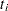is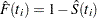, where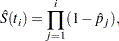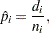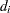is the number of failures in the interval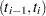, and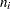is the number of unfailed units at the beginning of the interval. This definition of the Kaplan-Meier estimator is equivalent to the one previously given.

An estimator of the variance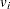of the Kaplan-Meier estimator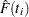is given by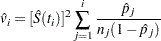An estimator of the standard deviation ofis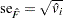.

For arbitrarily censored data, the Kaplan-Meier estimator is replaced by the nonparametric maximum likelihood estimator computed with the Turnbull algorithm, and the approximate variance of the estimator of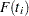is computed from the inverse of the Fisher information matrix.

### Pointwise Confidence Intervals

Approximate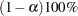pointwise confidence intervals are computed as in Meeker and Escobar (1998, section 3.6) as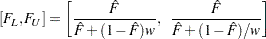where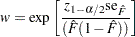where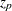is the
th quantile of the standard normal distribution.

### Simultaneous Confidence Intervals

Approximatesimultaneous confidence bands valid over the lifetime interval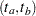are computed as the "Equal Precision" case of Nair (1984) and Meeker and Escobar (1998, section 3.8)where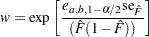where the factor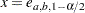is the solution of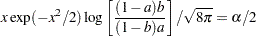The time intervalover which the bands are valid depends in a complicated way on the constantsanddefined in Nair (1984),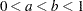.andare chosen by default, so that the confidence bands are valid between the lowest and highest times corresponding to failures in the case of multiply censored data, or, to the lowest and highest intervals for which probabilities are computed for arbitrarily censored data. You can optionally specifyanddirectly with the NPINTERVALS=SIMULTANEOUS(,) option in the PROBPLOT statement.Previous Page | Next Page | Top of Page